# 概揽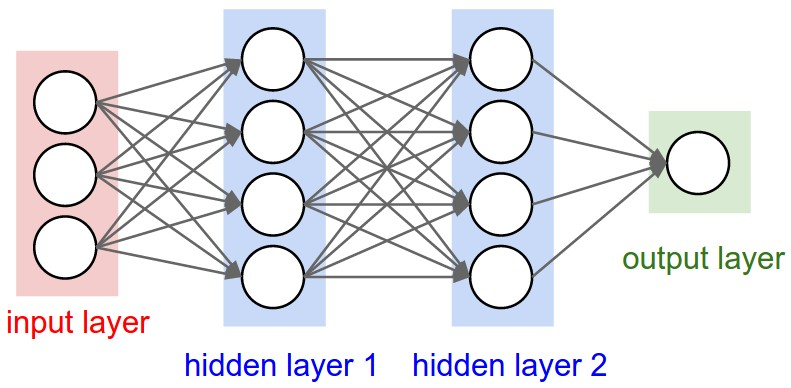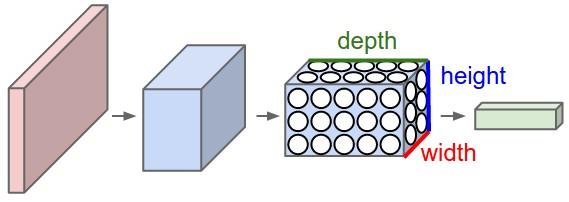# Layers used to build ConvNets

• 卷积层（Convolutional layer），卷积神经网路中每层卷积层由若干卷积单元组成，每个卷积单元的参数都是通过反向传播算法优化得到的。卷积运算的目的是提取输入的不同特征，第一层卷积层可能只能提取一些低级的特征如边缘、线条和角等层级，更多层的网络能从低级特征中迭代提取更复杂的特征。
• 线性整流层（Rectified Linear Units layer, ReLU layer），这一层神经的活性化函数（Activation function）使用线性整流（Rectified Linear Units, ReLU） f(x)=max(0,x) $f(x) = max(0, x)$
• 池化层（Pooling layer），通常在卷积层之后会得到维度很大的特征，将特征切成几个区域，取其最大值或平均值，得到新的、维度较小的特征。
• 全连接层（ Fully-Connected layer）, 把所有局部特征结合变成全局特征，用来计算最后每一类的得分。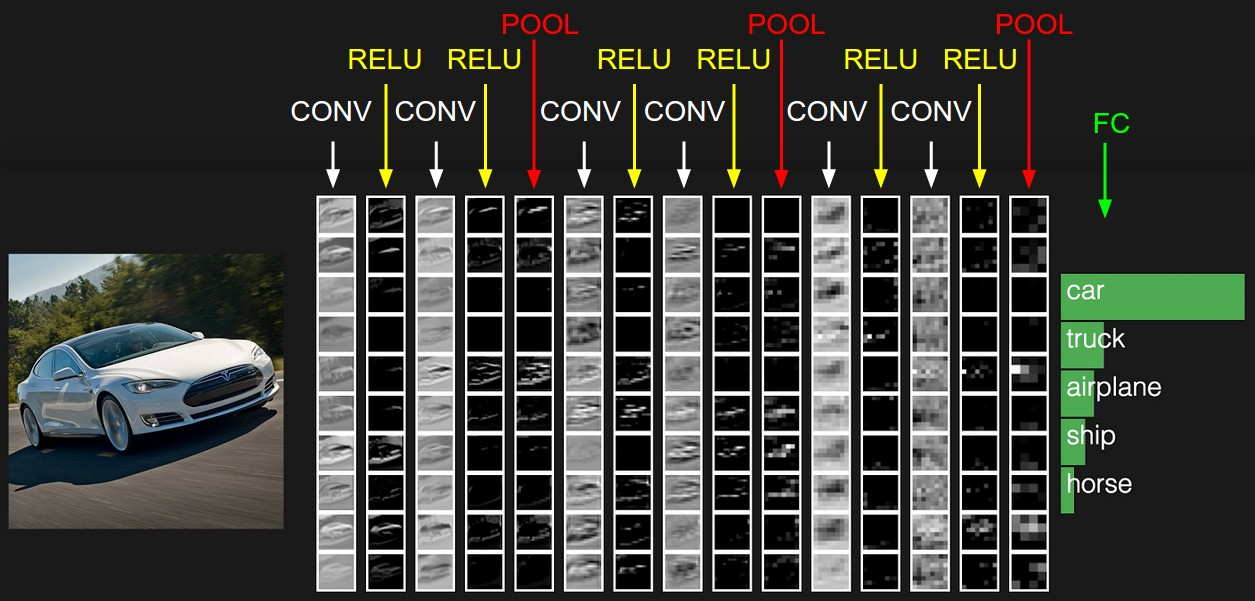## 卷积层（Convolutional layer）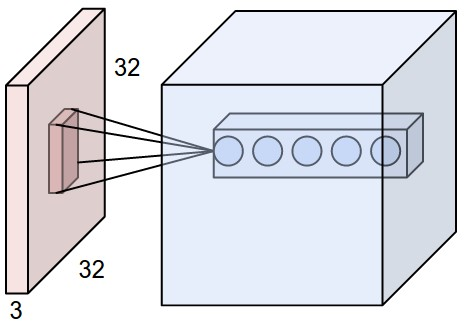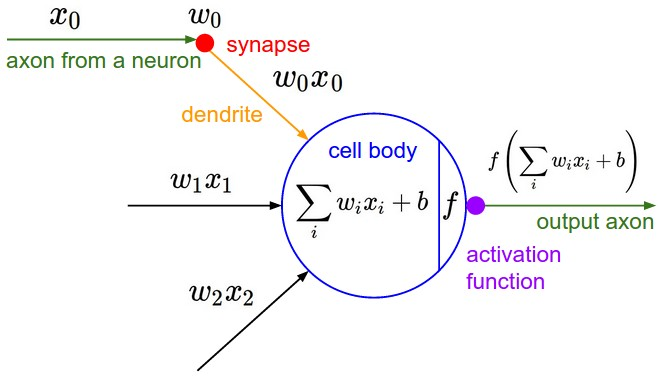• 深度(depth) : 顾名思义，它控制输出单元的深度，也就是filter的个数，连接同一块区域的神经元个数。又名：depth column
• 步幅(stride)：它控制在同一深度的相邻两个隐含单元，与他们相连接的输入区域的距离。如果步幅很小（比如 stride = 1）的话，相邻隐含单元的输入区域的重叠部分会很多; 步幅很大则重叠区域变少。

• W $W$ : 输入单元的大小（宽或高）
• F$F$ : 感受野(receptive field)
• S $S$ : 步幅（stride）
• P$P$ : 补零（zero-padding)的数量
• K $K$ : 深度，输出单元的深度

WF+2PS+1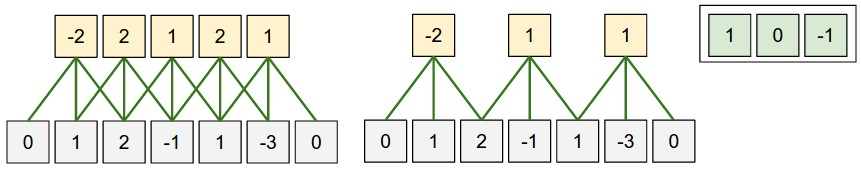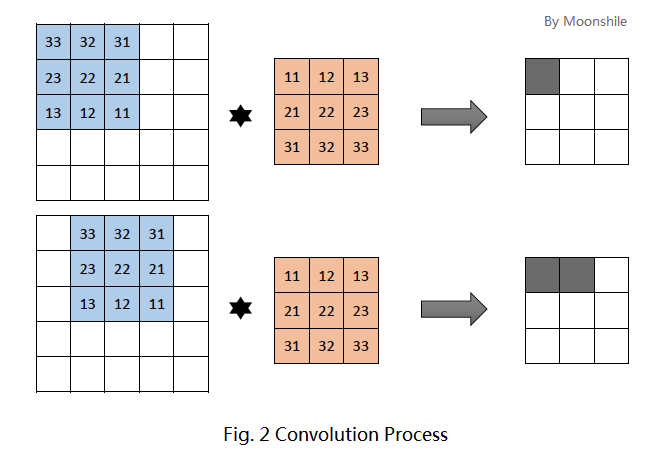f(x)=act(i,jnθ(ni)(nj)xij+b)

f(m,n)g(m,n)=uvf(u,v)g(mu,nv)

Numpy examples

* 位于 (x, y) 的 depth column 是 X[x, y, :]
* 深度为 d 的 depth slice 是 X[:, :, d]

• V[0,0,0] = np.sum(X[:5,:5,:] * W0) + b0
• V[1,0,0] = np.sum(X[2:7,:5,:] * W0) + b0
• V[2,0,0] = np.sum(X[4:9,:5,:] * W0) + b0
• V[3,0,0] = np.sum(X[6:11,:5,:] * W0) + b0

• V[0,0,1] = np.sum(X[:5,:5,:] * W1) + b1
• V[1,0,1] = np.sum(X[2:7,:5,:] * W1) + b1
• V[2,0,1] = np.sum(X[4:9,:5,:] * W1) + b1
• V[3,0,1] = np.sum(X[6:11,:5,:] * W1) + b1
• V[0,1,1] = np.sum(X[:5,2:7,:] * W1) + b1
• V[2,3,1] = np.sum(X[4:9,6:11,:] * W1) + b1

• 接收三维输入 W1H1D1 $W_1 * H_1 * D_1$
• 需要给出4个参数（hyperparameters）：
• Number of filters K $K$,
• their spatial extent F$F$,
• the stride S $S$,
• the amount of zero padding P$P$.
• 输出一个三维单元 W2H2D2 $W_2 * H_2 * D_2$，其中：
• W2=W1F+2PS+1 $W_2 = \frac{W_1 - F + 2P}{S} + 1$
• H2=H1F+2PS+1 $H_2 = \frac{H_1 - F + 2P}{S} + 1$
• D2=K $D_2 = K$
• 应用权值共享，每个filter会产生 FFD1 $F * F * D_1$ 个权重，总共 (FFD1)K $(F * F * D_1) * K$ 个权重和 K $K$ 个偏置。
• 在输出单元，第d个深度切片的结果是由第d个filter 和输入单元做卷积运算，然后再加上偏置而来。

## 池化层(Pooling Layer)

* 最大池化（Max Pooling）。取4个点的最大值。这是最常用的池化方法。
* 均值池化（Mean Pooling）。取4个点的均值。
* 高斯池化。借鉴高斯模糊的方法。不常用。
* 可训练池化。训练函数 ff ，接受4个点为输入，出入1个点。不常用。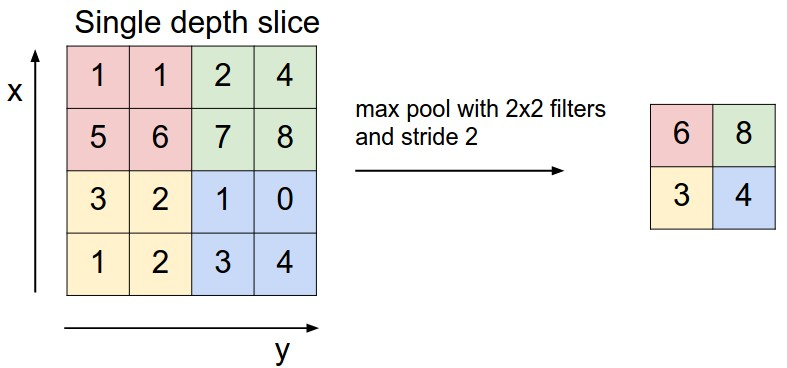• 接收单元大小为：W1H1D1$W_1 * H_1 * D_1$
• 需要两个参数（hyperparameters）：
• their spatial extent F $F$,
• the stride S$S$,
• 输出大小： W2H2D2 $W_2 * H_2 * D_2$，其中：
• W2=W1FS $W_2=\frac{W_1−F}{S}$
• H2=H1FS+1 $H_2=\frac{H_1−F}{S}+1$
• D2=D1 $D_2=D_1$
• 不需要引入新权重

## 全连接层（Fully-connected layer）

* 对于任意一个卷积层，要把它变成全连接层只需要把权重变成一个巨大的矩阵，其中大部分都是0 除了一些特定区块（因为局部感知），而且好多区块的权值还相同（由于权重共享）。
* 相反地，对于任何一个全连接层也可以变为卷积层。比如，一个 K4096 $K ＝ 4096$ 的全连接层，输入层大小为 77512 $7*7*512$，它可以等效为一个 F=7, P=0, S=1, K=4096 $F=7,\ P=0,\ S=1,\ K=4096$ 的卷积层。换言之，我们把 filter size 正好设置为整个输入层大小。

# 卷积神经网络架构

## Layer Patterns

INPUT -> [[CONV -> RELU]*N -> POOL?]*M -> [FC -> RELU]*K -> FC

* INPUT -> FC, 实现了一个线性分类器， 这里 N = M = K = 0
* INPUT -> CONV -> RELU -> FC
* INPUT -> [CONV -> RELU -> POOL]*2 -> FC -> RELU -> FC. Here we see that there is a single CONV layer between every POOL layer.
* INPUT -> [CONV -> RELU -> CONV -> RELU -> POOL]*3 -> [FC -> RELU]*2 -> FC Here we see two CONV layers stacked before every POOL layer. This is generally a good idea for larger and deeper networks, because multiple stacked CONV layers can develop more complex features of the input volume before the destructive pooling operation.

## Layer Sizing Patterns

• Input layer : 应该是2的整数次幂。比如32，64， 128等。
• Conv Layer : 使用小的过滤器（filter）， F=3 or F=5 $F = 3\ or\ F = 5$, 步幅 S=1 $S=1$，如果不能恰好拟合输入层，还要边缘补零。如果使用 F=3, P=1 $F = 3,\ P = 1$，那么输出大小将与输入一样。如果用更大的过滤器（比如7*7），一般只会在紧挨着原始输入图片的卷积层才会看到。
• Pool Layer : F=2, S=2 $F = 2,\ S = 2$

## Case Studies

• LeNet. The first successful applications of Convolutional Networks were developed by Yann LeCun in 1990’s. Of these, the best known is the LeNet architecture that was used to read zip codes, digits, etc.
• AlexNet. The first work that popularized Convolutional Networks in Computer Vision was the AlexNet, developed by Alex Krizhevsky, Ilya Sutskever and Geoff Hinton. The AlexNet was submitted to the ImageNet ILSVRC challenge in 2012 and significantly outperformed the second runner-up (top 5 error of 16% compared to runner-up with 26% error). The Network had a similar architecture basic as LeNet, but was deeper, bigger, and featured Convolutional Layers stacked on top of each other (previously it was common to only have a single CONV layer immediately followed by a POOL layer).
• ZF Net. The ILSVRC 2013 winner was a Convolutional Network from Matthew Zeiler and Rob Fergus. It became known as the ZFNet (short for Zeiler & Fergus Net). It was an improvement on AlexNet by tweaking the architecture hyperparameters, in particular by expanding the size of the middle convolutional layers.
• GoogLeNet. The ILSVRC 2014 winner was a Convolutional Network from Szegedy et al. from Google. Its main contribution was the development of an Inception Module that dramatically reduced the number of parameters in the network (4M, compared to AlexNet with 60M). Additionally, this paper uses Average Pooling instead of Fully Connected layers at the top of the ConvNet, eliminating a large amount of parameters that do not seem to matter much.
• VGGNet. The runner-up in ILSVRC 2014 was the network from Karen Simonyan and Andrew Zisserman that became known as the VGGNet. Its main contribution was in showing that the depth of the network is a critical component for good performance. Their final best network contains 16 CONV/FC layers and, appealingly, features an extremely homogeneous architecture that only performs 3x3 convolutions and 2x2 pooling from the beginning to the end. It was later found that despite its slightly weaker classification performance, the VGG ConvNet features outperform those of GoogLeNet in multiple transfer learning tasks. Hence, the VGG network is currently the most preferred choice in the community when extracting CNN features from images. In particular, their pretrained model is available for plug and play use in Caffe. A downside of the VGGNet is that it is more expensive to evaluate and uses a lot more memory and parameters (140M).
• ResNet. Residual Network developed by Kaiming He et al. was the winner of ILSVRC 2015. It features an interesting architecture with special skip connections and features heavy use of batch normalization. The architecture is also missing fully connected layers at the end of the network. The reader is also referred to Kaiming’s presentation (video, slides), and some recent experiments that reproduce these networks in Torch.

# 参考